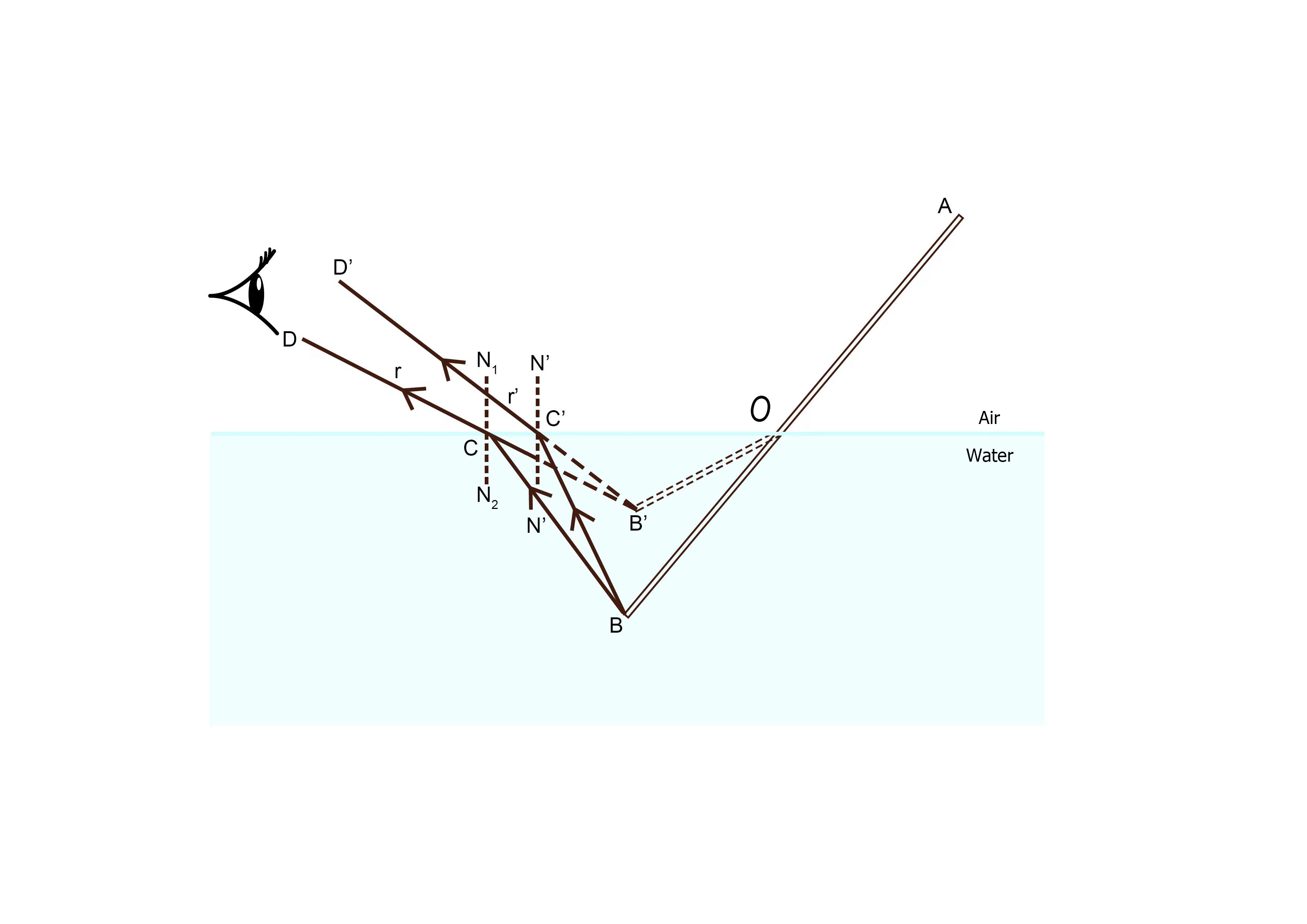## Lesson 4 – How Does Light Travel? Part II: Refraction

Posted on: Friday, February 4th, 2022  In: Learning Optics with Austin

Why does a pencil look like it is snapped in half when partially placed in a container of water? The short answer is refraction.Pencil Demonstration of Refraction

A more detailed explanation is that the rays of light bends when they pass from one medium to another. This bending of light from air to water produces a virtual image of the pencil in water that is different from the pencil’s actual position.Refraction Diagram

The way we measure refraction is through the refractive index (n). We define ⍺ to be the angle of incidence and Ɣ to be the angle of refraction. By the relationship sin(⍺) / sin(Ɣ) = n0 / n1, the refractive index (n) = n0 / n1. Thus, the larger n is, the smaller the angle of refraction which also means a greater bending of light.Equation for Refractive Index

While it is the case when light travels from air to water that the angle of refraction is smaller than the angle of incidence, this is not always true. In fact, if light were to travel from water to air, then the angle of incidence would be greater than the angle of refraction. What is always true though, is that the angle of incidence is equivalent to the angle of reflection. This brings us back to the topic of reflectivity and transmission covered last week.Reflection, Refraction, and Transmission Combined

Not all light that hits a surface goes through refraction. Depending on the conditions of the surface, light is also reflected. This is why the equation for reflectivity and transmission adds up to 100%. Thus, a material with high reflectivity will have low transmission and vice versa. Reflectivity and transmission are two crucially related variables that are considered in the designing of optical components.

Next: Lesson 5 – Intro to Optical Components